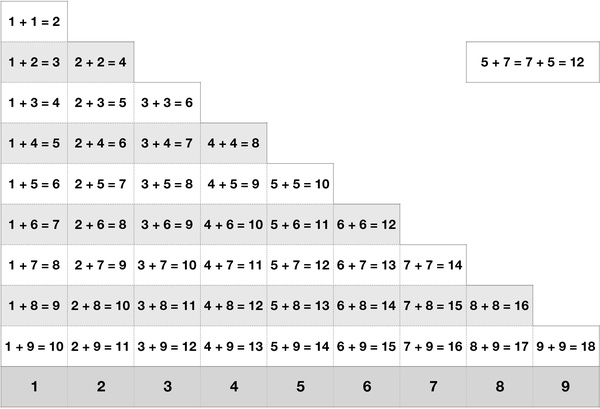# Addition Table 9 by 9Addition, subtraction, multiplication and division are the four elementary mathematical operations of arithmetic. Around 6 years old, most kids are ready to learn facts about addition. Addition is the first operation kids learn. Mastery of the small number addition facts is the foundation for learning other elementary arithmetic operations. In mathematics, an addition table is a mathematical table used to define an addition operation for numbers.

This worksheet contains a chart of 45 multiplication results of factors from 1 to 9. This resembles the Chinese multiplication table on multiplication equations, making it easy for children to learn by heart. Duplicates are eliminated by applying the commutative property. Memorizing the addition table is an important skill for learning mathematics. With the help of this table, parents could help kids learn, memorize, practice and recall the facts of adding small numbers easily. Once kids master this skill, they are well-prepared to tackle more advanced math topics such as multi-digit addition and multiplication. It is also useful to explore patterns on the table to help kids memorize the facts.